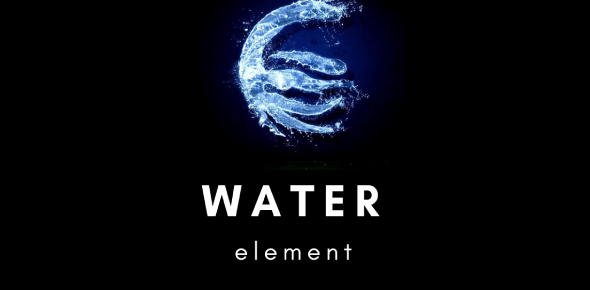Knowledge Test: Element Of Water! Trivia Quiz

10 Questions | Total Attempts: 61670SettingsDo you know which two elements make up water? Do you know how long the average human can survive without water? Take this quiz to find out the answers to these questions, amongst others! Good luck!

• 1.
Which two elements make up water?
• A.

Hydrogen and oxygen

• B.

Sodium and calcium

• C.

Calcium and oxygen

• D.

Hydrogen and sodium

• 2.
Sound travels faster through water than air.
• A.

True

• B.

False

• 3.
How long can the average human survive without water?
• A.

1 day

• B.

A few days

• C.

A few weeks

• D.

A month

• 4.
At what temperature does water boil?
• A.

80 C

• B.

100 C

• C.

150 C

• D.

200 C

• 5.
When water is cooled, does it contract or expand?
• A.

Contract

• B.

Expand

• 6.
At what temperature does water freeze?
• A.

- 10 C

• B.

0 C

• C.

5 C

• D.

10 C

• 7.
What is the average pH level of pure water?
• A.

3

• B.

5

• C.

7

• D.

9

• 8.
In which step of the water cycle does liquid water transform into gas?
• A.

Condensation

• B.

Precipitation

• C.

Transpiration

• D.

Evaporation

• 9.
What percentage of the Earth's water supply is fresh water?
• A.

3%

• B.

9%

• C.

15%

• D.

20%

• 10.
What property of water allows it to stick to itself?
• A.

• B.

Coagulation

• C.

Cohesion

• D.

Condensation

Related TopicsBack to top